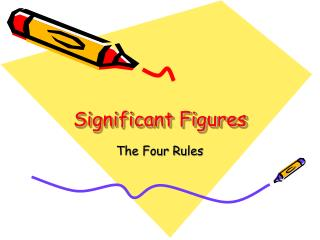DownloadDownload PresentationSignificant Figures

# Significant Figures

Télécharger la présentation## Significant Figures

- - - - - - - - - - - - - - - - - - - - - - - - - - - E N D - - - - - - - - - - - - - - - - - - - - - - - - - - -
##### Presentation Transcript

1. Significant Figures The Four Rules

2. Rule #1:All non-zero numbers are always… SIGNIFICANT!!! 4 3 6 9 2 7 8 1 5

3. Using Othello to understand Rule #2 Zeros between non-zero numbers are always significant

4. Using Othello to understand Rule #2 Zeros between non-zero numbers are always significant

5. The Other Zero Rules Rule #3: All final zeros to the ______ of a decimal point are significant. right 0.0000340000 Rule #4: Zeros that act as ____________ are NOT significant. placeholders

6. For rule #3 and #4, it can be summarized by these two questions: 1) Is there a decimal point? 2) Is there a number in front of the zero? Answering yes to both of these questions means that the zeros are significant figures.

7. Significant? 1000000 .0

8. Another note: if a number is written in scientific notation, all numbers before the x 10 are significant, all numbers after are not significant.

9. Is ItSignificant?

10. Significant Non-Significant 16407.100 8 sig figs 0.00010080 5 sig figs 904008000 6 sig figs

11. Let’s look at some examples a. 508.0 L b. 820 400.0 L c. 1.0200 x 105 kg d. 807 000 kg 4 significant figures 7 significant figures 5 significant figures 3 significant figures

12. Let’s look at some examples a. 0.049 450 s b. 0.000 482 mL c. 3.1587 x 10-8 g d. 0.0084 mL 5 significant figures 3 significant figures 5 significant figures 2 significant figures

13. So, why are significant figures important? Significant figures and math

14. 187.6 961.95 + 2.303 - 943 Addition and Subtraction • When adding or subtracting—the answer has the least number of decimal places • Hint: Before adding and subtracting, line up all the numbers so the decimal points align. 189.903 18.95 189.9 19

15. 21.6 7216 x 0.067 ÷ 0.034 Multiplication and Division • When multiplying or dividing—the product has the least number of significant figures (3 sig figs) (4 sig figs) (2 sig figs) (2 sig figs) 1.4472 212235.294118 (2 sig figs) (2 sig figs) 1.4 210000 Or 2.1 x 105

16. Now, try some of these on your own

17. a) 43.2 cm 51.0 cm + 48.7 cm b) 258.3 kg 257.1 1 kg + 253 kg c) 0.0487 mg 0.05834 mg + 0.00483 mg 768 kg 0.1119 mg Addition 768.41 kg 0.11187 mg 142.9 cm

18. Multiplication a) 24 m x 3.26 m = 78.24 m = 78 m b) 120 m x 0.10 m = 12 m c) 1.23 m x 2.0 m = 2.46 m = 2.5 m d) 53.0 m x 1.53 m = 81.09 m = 81.1 m

19. Why do we have them? When we measure things, we want to measure to the place we are sure of and guess one more space.

20. So, they show the uncertainty in our measurements

21. Since the marks on this ruler are subdivided as they are, our answer for the length of this nail has 3 significant figures.

22. However, we only have significant figures when we are measuring something. Counting will give you an exact number.